# Recent Progress of SIS Calibration and Software

## T. Dotani1, A. Yamashita1, H. Ezuka1, K. Takahashi1 G. Crew2, K. Mukai3, and the SIS team

### 1: ISAS 2: MIT 3: NASA/GSFC

dotani@astro.isas.jaxa.jp

1 Introduction

Three topics are reported on the recent progress of SIS calibration and software. The parameters of the charge transfer efficiency (CTE) have been rederived to minimize the systematic errors in the SIS gain using the Cas A data obtained up to 1996 and the Ni line in the background. We have traced the history of the energy resolution degradation due to the non-uniform CTE, and found that the empirical formula currently in use is still valid for the latest data. Finally, the development of the software and database used to reduce the RDD effects is described.

2 Charge Transfer Efficiency

It is known that the charge transfer efficiency (CTE) of the CCDs used in ASCA SIS is gradually decreasing due to the radiation damage [3, 6]. The decrease of the CTE, or in other words, the increase of the charge transfer inefficiency (CTI), causes the loss of charge during the charge transfer. This reduces the apparent X-ray energy and hence brings about a reduction of gain. The average reduction of the X-ray energy due to the CTI can be predicted, and may be restored in the course of data reduction. The CTI values currently in use (released on 2/29/96) were determined mostly based on the Cas A data in 1995 and before . However, because the CTI corrected data were found to show a gradual change of the gain, we have rederived the CTI values using all available Cas A data and the Ni data in the background.

The CCDs in ASCA SIS use 3 kinds of clocks to transfer charge packets from the imaging region to the read-out gate in the normal mode; the clocks are ST (store) clock, the parallel clock and the serial clock. ST clock transfers all the charges accumulated in the imaging region to the frame-store region. Note that the number of transfer by ST clock is the same for all pixels. The parallel clock transfers the charges in the frame-store region to the serial register row by row, and the serial clock transfers charges in serial register to the read-out gate pixel by pixel. These three kinds of clocks are schematically shown in figure 1. ST and the serial clocks are relatively fast, but the parallel clock is slow. Because the CTI depends on the speed of the charge transfer, CTI associated with these 3 clocks may be different. Thus, we need to determine the CTI for these 3 clocks separately.

Figure 1 Direction of charge transfer by ST (store) clock, the parallel clock and the serial clock are schematically shown. The CTI needs to be determined for these clocks separately. Pointing positions during the Cas A observations are also indicated with filled circles with labels.

We have used two sets of data to determine the CTI values, the Ni line in the background and the Cas A data. The Ni line data are basically used to investigate the time dependence of the average CTI, and the Cas A data are used to decompose the 3 kinds of CTI.

2.1 Ni line in the background

The Ni line data were accumulated from standard chips (SIS-0 chip-1 and SIS-1 chip-3) in 1 ccd faint mode. We accumulated all the available data regardless of the presence of X-ray sources in the fov unless they are bright (>0.1 cts/sec). This is because background count rate exceeds that of the X-ray sources above ~ keV unless they are bright, and presence of the dim X-ray sources does not affect the determination of the Ni line energy. We determined the center energy of the Ni line every 3 months;the results are shown in figure 2. The data have been corrected for echo and DFE, but not for the CTI. Degradation of the energy resolution mostly due to the non-uniform CTI was included in the response. The Ni events are generated in both imaging and frame-store regions, and the number of transfers is not known even if the (h, v) address of the event is specified. This means that we cannot apply the CTI correction directly to the event data as is usually done.

The gradual decrease of the Ni line energy clearly seen in the figure is due to the increase of CTI. The best-fit linear function to the data is also shown in the figure. The decrease of the line energy is well approximated by a linear function of time. Because the parallel and the serial CTIs are consistent with zero at launch according to the analysis of Cas A data, this indicates that the CTI increases linearly with time. Although this only means that a combination of 3 kinds of CTI results in a linear trend, it may be natural to consider that all 3 CTIs increase linearly with time. Hereafter, we assume that the CTIs increase in proportion to time after the launch, although the rate of increase may be different for different clocks.

2.2 Cas A data

Cas A observations are carried out once a year for the calibration of SIS. Although the number of pointings were relatively small in early observations, we observed Cas A at 4 different pointing positions on the standard chips in 1995 and 1996. The pointing positions are indicated in figure 1. In principle, 4 pointings are more than enough to distinguish parallel and serial CTI, if both CTIs are constant over the chip. However, we know that CTI is not uniform over the chip, and this non-uniformity makes it very complicated to determine the appropriate CTI values. We used systematic difference of the line energies between the pointing positions to determine the CTIs in the previous analysis. However, this method seems to be sensitive to the local value of CTI and not reflect the global value. A better method may be to determine the CTI to give consistent line energies among different pointing positions and observation epochs.

Figure 2 The long-term history of the apparent center energy of the Ni line in the background. The data used are 1 ccd faint mode, and have been corrected for echo and DFE, but not for CTI. Degradation of the energy resolution is included in the response. The gradual decrease of the apparent Ni line energy is due to the increase of the CTI. The best-fit linear function is also plotted in the figure. The decrease of the line energy, or equivalently the increase of the CTI, is well approximated to be proportional to the time after the launch of the satellite.

We show in figure 3 the apparent center energies of the prominent emission lines (Si, S and Fe) in the energy spectrum of Cas A for different pointing positions as a function of the observation time. The data are corrected for DFE, echo and CTI. The calibration file we used to correct the CTI is the latest one (sisph2pi_290296.fits). It is clear from the figure that the line energies show significant change even after the CTI/echo/DFE corrections. There is a tendency that the line energies at the pointing position 'x' are systematically different from other data sets. This may be because the CTI values currently in use were determined from the data obtained at 'o', 'h' and 'v', and data from 'x' were not used. This long-term variations of the line energies are considered to result from the fact that the CTI values we used do not reflect the global values correctly. Thus, we rederived the CTI values to give almost constant and consistent line energies in the chip.

In adjusting the CTI, we have assumed that the CTI is proportional to the time after the launch of ASCA. This assumption means that CTI increases with a constant rate after the launch, but the rate may be different for different chips and clocks ([[section]]1.1). We, therefore, adjust not CTI itself but the CTI increase rate. The adjustment will be done independently for SIS-0 and SIS-1. We further assume that CTI is constant against X-ray energy. Thus, a constant fraction of charge is assumed to be lost by the CTI from the charge packets regardless of their size. This assumption has already been incorporated in the current SIS calibration file (sisph2pi_290296.fits). We are aware that this assumption may not be correct: There is a tendency for the fraction of charge loss to become smaller for larger charge packets. Further analysis is needed to determine the appropriate formula for this energy dependence of the CTI, which may be incorporated in the analysis software in future (See [[section]]4). Some of the pointing positions of the Cas A observations (e.g.. position 'o') is close to the nominal pointing position of 1 ccd mode, but some (e.g.. position 'x') are far away. Thus, we have placed higher weights on the position 'o' data to adjust the CTI increase rates.

Figure 3 The center energies of the prominent emission lines in the Cas A energy spectra. The data used are 1 ccd faint mode corrected for echo, DFE and CTE. Different symbols indicate different pointing positions (see figure 1). Circles (and solid line) indicate pointing position 'o', squares (and broken line) 'h', triangles (and dotted line) 'v', and crosses (and dot broken line) 'x'. Long-term change of the line energies and their differences at different pointing positions are considered mostly to be due to the in accurate CTI values currently in use.

Table 1: CTI increase rates for the standard chips
```__________________________________________________________________________________________________
S0C1						 S1C3
ST 		Parallel		Serial		  ST		Parallel		Serial
__________________________________________________________________________________________________

Present values*		0.956		1.611	 	2.155           1.765		1.660		-0.160
Revised values		1.406		1.811		2.505		1.615		1.760		 0.540
__________________________________________________________________________________________________
Values listed are in unit of 10-5 transfer-1yr-1.
```

The Si line energies obtained with SIS-1 in 1996 show a slightly different trend than other data. They seem to be a little higher than extrapolated values. Although the reason of this different trend is not known yet, we suspect that optical light leak seen only in SIS-1 on rare occasions may be related to this shift of the apparent line energy (this is a different phenomenon from the light leak seen in SIS-0 chips 2 and 3). The extra charges generated by optical light tend to fill the charge traps, and reduce the number of electrons lost in the course of charge transfer. Although this may be a possible explanation, we do not understand yet how optical light comes into only SIS-1. For the present analysis, we do not use the Si line energies of SIS-1in 1996.

We list the adjusted CTI increase rates in table 1 with those currently in use (sisph2pi_290296.fits). The history of the prominent line energies in Cas A with these new CTI increase rates is shown in figure 4. A scatter of the line energies may be still present, but their long-term gradual changes are much reduced. The scatter of the line energies among the pointing positions is considered to be due to the non-uniformity of the CTI. This causes degradation of the energy resolution, which will be discussed in [[section]]2. A revised table of CTI values is expected to be released by the time this articles is in print.

Figure 4 Same as figure 3, but the revised values are used for CTI (see table 1).

The present analysis also indicates the systematic error of the energy scale. The inconsistency of the line energies among the pointing positions and observation epoch after the CTI corrections should be regarded as systematic error in the energy scale. The systematic error in the energy scale is estimated to be 0.3% at the energy of Si line and 0.5 % at the energy Fe line. The larger systematic error at higher energies may result from the assumption that the CTI is independent of X-ray energy. The systematic error may also be checked with the Ni line data. If we use the revised CTI values in table 1, the apparent Ni line energies are expected to decrease 1.22% yr for S0C1 and 0.85% yr for S1C3, respectively. On the other hand, observed decrease rates of the apparent Ni line energy are 1.2 pm 0.1 % yr for S0C1 and 0.6 pm 0.1 yr for S1C3, respectively. Thus, the calculated and observed decrease rates coincide for S0C1, but are different for S1C3. In addition, there may be a slight discrepancy in the energy scale between S0C1 and S1C3 by about 0.5%. The discrepancy may be recognized in both the Cas A data and the Ni line data. Because the absolute gain of S0C1 was adjusted to give correct energy for Ni line, we believe that S1C3 shows systematically lower gain. When we determine the relative gain between chips, we used CTI corrected data. This means that relative gain depends on the CTI values. Now we have revised the CTI values, the relative gain need to be adjusted once again. See [[section]]4 for the analysis planned to recalibrate the relative gain.

3 Energy resolution

It is known that the energy resolution of SIS is gradually degrading due to the RDD and the non-uniform CTI. In the case of 1 ccd mode, non-uniform CTI is the main source of energy resolution degradation, while both the RDD and the non-uniform CTI contribute in the case of 2/4 ccd modes (see [[section]]3). As reported previously, the degradation of the energy resolution due to the non-uniform CTI can be modeled empirically as a change of the Fano factor. We investigated whether or not this empirical formula is a good approximation even for the latest data.

The empirical formula of the Fano factor (f) is:

`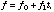(1)`

where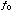is the Fano factor at launch time,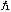Fano factor variation coefficient ( 3.3 x 10-9 for SIS-0 and 2.9 x 10-9 for SIS-1), time after the launch (sec). With this model of the Fano factor, apparent line width (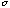in eV)

of Cas A is expected to change as follows:

`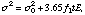(2)`

where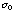is the intrinsic line width (eV),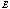line energy (eV).

Figure 5 The apparent widths of the 3 prominent emission lines in Cas A energy spectra. The data used are in 1 ccd faint mode obtained at position 'o' (see figure 1), and are corrected for echo, DFE and CTI. The increase of the apparent line width is due to the non-uniformity of the CTI, which results in the degradation of the energy resolution. The empirical formula of the degradation of the energy resolution is used to reproduce the apparent line width. The data obtained so far can be represented well with this formula.

We show in figure 5 the history of the apparent line widths of Cas A. The data used are in 1 ccd faint mode obtained at position 'o'. The data are corrected for echo, DFE and CTI. The same response matrices, which correspond to that at the launch time, is used to fit the energy spectra of all the data sets. The model line widths plotted in the figure are those determined with the data up to 1995. Thus, the data in 1996 just stay on the extrapolation of the model. This means that the empirical model of the energy resolution degradation currently in use is still good enough for the analysis of the latest data.

We used data from the pointing position 'o' to obtain the above results. Caution should be paid when the data obtained at the pointing position very different from 'o' (effectively 1 ccd nominal position) are analyzed. Charge loss due to CTI is proportional to the number of charge transfer. When the number of transfer is relatively small (e.g. the case of pointing position 'x'), variation of the charge loss due to non-uniform CTI would also become small. This means that energy resolution degradation may be small when the number of charge transfer is small. Such position dependence of the energy resolution degradation is not incorporated in the formula currently in use. Although this effect may be noticeable only when the pointing position is very different from 'o' and the statistics of the data is very high, those who analyze the SIS data of non-standard pointing position should be aware of this effect.

4 RDD correction

In 4/2 ccd modes, the RDD effects causes further degradation of the CCD performance in addition to the non-uniform CTI. However, the RDD effects may be reduced largely in the course of data processing provided that appropriate calibration data are available. The principle of the RDD correction is explained in this section with the demonstration using the Cas A data.

The RDD results from the increase of the dark current due to the radiation damage. We have found that the increase of the dark current is very different from pixel to pixel, although the dark current does not show a large variation on a global scale within a chip. On-board data processing assigns only a single dark level for 16x16 pixels due to a limited computer resources. Thus, the pixel-to-pixel variations of the dark current cannot be corrected for on board. This excess variation is called the RDD (Residual Dark Distribution) and causes various effects on the SIS performance, such as a degradation of the energy resolution, a decrease of the detection efficiency, and so on. Because the dark current is larger for longer exposure, the RDD effects are most significant in 4 ccd mode (16 sec exposure) and are still negligible in 1 ccd mode (4 sec exposure). Thus, the RDD correction should be effective only for the data of 2/4 ccd modes.

Although the average dark current increases gradually with time, the dark current of an individual pixel shows quite a different behavior. It is stable for most of time (within the read-out noise), but shows abrupt change a few times a year. The abrupt change seems to occur during the SAA passage of the satellite. This means that we can define a so-called dark current map which is a good approximation of the true dark currents for a few months. Such a dark current map can be calculated from the frame mode data, in which pulse height of all the pixels are dumped to the telemetry.

Instead of using the dark current map directly, we use an RDD map calculated as follows for the RDD correction.

1. Take several sets of frame mode data.

2. Subtract the bias level, and then subtract the dark level calculated every 16x16 pixel region.
Because the dark level is defined for the image which is subtracted for the dark level, we need iteration of several times for the dark level to converge.

3. Take the average of the images obtained in step 2 by removing pixels contaminated by charged particles or X-ray photons.

4. Adjust the offset of the image to have a zero mean.

These steps, although not exactly the same, emulate the on-board data processing. The image thus obtained represents the deviation of the dark currents from the local mean (defined every 16x16 pixel region), and is referred to as the RDD map. Note that we need to calculate the RDD map for each pair of chip and exposure time.

Using the RDD map, we can correct the pixel-to-pixel variations of the dark current for faint mode data. Method of RDD correction is very similar to the DFE correction. In the case of DFE correction, a constant value (= DFE) is subtracted from the pulse height of each pixel, whereas a value assigned to each pixel (= RDD map) is subtracted from the corresponding pixel for the RDD correction. RDD correction should be effective only for the faint mode data. RDD correction restores a major part of the degradation of the energy resolution and the decrease of the detection efficiency. Example of the RDD correction is shown in figure 6.

Figure 6(a) shows the energy spectrum obtained from Cas A with SIS-0 chip-1 in 4 ccd mode in August 1996 using only the single event data (grade 0). The data were taken in faint mode, thus the echo, CTE and DFE corrections were applied to the data. If we further apply the RDD correction, we obtain the energy spectrum in figure 6(b). For the RDD correction, we used the RDD map calculated from the frame mode data obtained near the Cas A observations. A significant improvement in the energy resolution and the detection efficiency is clearly seen in the figure. We show in figure 6(c) the energy spectrum obtained in 1 ccd mode at the same epoch for comparison. It is seen from the figures that the RDD correction restores the energy resolution and the detection efficiency of 4 ccd mode data close to those of 1 ccd mode, although a small difference (~10 %) in the detection efficiency seems to remain. Figure 6(d) shows the pulse height distribution of the corner pixels of single event data. The corner pixels of a single event (grade 0) are usually free of charges generated by X-rays and hence their pulse height is considered to represent the deviation of the dark current of the pixel from a local mean. The 4 ccd mode data (no RDD correction) show a very wide and asymmetric distribution; this is the cause of degradation of the energy resolution and the detection efficiency. When we apply the RDD correction, the distribution becomes narrow and symmetric close to that in 1 ccd mode data. This is the reason why the energy resolution and the detection efficiency are restored by the RDD correction. There are still some differences in the corner pixel distribution between 1 ccd mode data and the RDD corrected 4 ccd mode data. This may be the reason why the RDD correction does not restore the detection efficiency completely. Except for the small difference in the detection efficiency, RDD corrected 4 ccd mode data may be analyzed as if they were 1 ccd mode data.

SIS team and GSFC are working to release the software and database necessary for RDD correction. Frame mode data, from which the RDD maps are created, are taken typically several times a month during the occultation of the X-ray sources by the night earth, if the on-board data recorder has enough free space. However, earth occultation's long enough to take the frame mode data is relatively infrequent and the number of frame mode data is not necessarily large enough to make the RDD maps every month. Thus, we recommend those who wish to apply the RDD correction to take frame mode data during their own observation time.

5 Future calibration

The SIS team is working continuously to improve various aspects of calibration of SIS. Analysis currently in progress which will be reflected in the calibration data in future is described below.

The CTI is considered to be in general a function of various parameters. When the CTI was relatively small, it was appropriate to make simple assumptions on the CTI such as uniformity, energy independence, 1/2/4 ccd mode independence, X-ray flux independence, etc. (see  for the X-ray flux dependence). However, the gradual increase of the CTI makes it necessary to introduce a more realistic functional from to the CTI model. We are going to introduce dependences on two parameters: an energy dependence and 1/2/4 ccd mode dependence.

It is assumed at present that the charge lost by CTI is proportional to the size of the charge packet. This means that the CTI is energy independent. It is considered in general that the charge loss due to the CTI increases with the size of the charge packet, but is smaller than the linear relation. In other words, the CTI is smaller for larger charge packets (higher energy X-rays). We are now studying appropriate functional form for the energy dependence of the CTI. The other dependence we are going to introduce is the 1/2/4 ccd mode (i.e. exposure) dependence of CTI. A preliminary analysis indicates that the CTI tends to decrease for longer exposures. This is considered to be due to the extra-charge of dark current and/or optical light leak. The extra charges fill some of the charge traps, and reduces the number of electrons lost during the charge transfer. This results in a smaller CTI.

Investigation of the realistic functional form to the CTI model will be followed by the readjustment of the relative gain. Relative gain between chips must be determined for the epoch oft satellite launch. However, all the flight data were obtained some time after the launch. This means that CTI should be incorporated correctly in the analysis of the relative gain. Note that CTI values are determined by the relative difference of the line energies and are independent of the gain. Hence, there is no need of iteration between the gain and the CTI. SNR with multiple pointings and the Ni line in the background will be used for this analysis.

We have so far placed emphasis on the calibration of faint/bright mode data, because they are most frequently used. However, analysis of fast mode data shows that this mode has different response from the faint/bright modes. The difference seems to be larger at higher energies. Furthermore, the CTI may also be different in the fast mode. Although the fast mode data available for calibration are rather limited, some of the Cas A data were obtained in fast mode. A preliminary analysis of these Cas A data has been done, but we need further work to make appropriate calibration files.

The low energy calibration is a continuing problem. We understand that this is a calibration issue related to all ASCA instruments (XRT, GIS and SIS), not SIS alone. In the early phase of ASCA calibration, XRT+GIS and XRT+SIS were calibrated to give consistent results with each other and with past missions. A re-calibration involving all three instruments is in progress (see the article by Gendreau and Yagoob 1997, ASCANEWS Volume 5), and the low energy response of the SIS will be investigated in the course of re-calibration.

6 Reference

Dotani, T. et al. 1995, ASCA news No. 3, 25
Dotani, T. et al. 1996, ASCA news No. 4, 3
Gendreau, K. C. 1995, PhD thesis, Massachusetts Institute of Technology
Gendreau, K. C. et al. 1995, IEEE trans. Electron Dev. 42, No. 11, 1912
Yamashita, A. 1995, Master thesis, The University of Tokyo (in Japanese)
Yamashita, A. et al. 1997, IEEE trans. Nucl. Sci., 44, No. 3, in press

Figure 6 An example of the RDD correction. (a) The energy spectrum of Cas A obtained with S0C1 in 4 ccd mode (16 sec exposure) in August 1996 using only the single event data (grade 0). Level discrimination was raised to 1.5 keV during the observations to prevent telemetry saturation. Data were obtained at the pointing position 'x'. (b) The RDD corrected energy spectrum. The data used are the same as for figure (a), but are corrected for RDD. Note that the energy resolution is better in RDD corrected spectrum and the detection efficiency is higher. (c) The energy spectrum of Cas A obtained with S0C1 in 1 ccd mode (4 sec exposure) at the same epoch and pointing position using only the single events (grade 0). A comparison of figure (b) and (c) shows that the RDD correction can restore 4 ccd mode data to the quality of 1 ccd mode data. (d) The pulse height distribution of the corner pixels of the event data before the RDD correction (dotted line) and after the RDD correction (broken line). The broad and asymmetric distribution before the RDD correction causes the degradation of the energy resolution and the detection efficiency. RDD correction makes the distribution narrow and symmetric like the one in 1 ccd mode (solid line).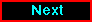Proceed to the next article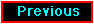Return to the previous article